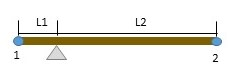# The figure shows particles 1 and, each with mass m, attached to the ends of a rigid massless rod...

## Question:

The figure shows particles 1 and, each with mass m, attached to the ends of a rigid massless rod of length L1 + L2, with L1 and L2 defined as shown. The rod is held horizontally on the fulcrum and then released. What are the magnitudes of the initial accelerations of (a) particle 1 and (b) particle 2? Note that L2 is greater than L1.## Torque:

When the body rotates about the fixed axis of rotation due to the external force acts on the body at a distance apart from the axis of rotation then it is called as torque. Both particles apply force mg vertically downwards. This mg force applies torque on the rod, and the point of a rod having zero velocity will be the fulcrum point. So, we will find the net torque about that point for calculations.

{eq}\text {Torque =Force} \times \text {Perpendicular distance between force and axis of rotation} \\ \tau=Fr {/eq}

Become a Study.com member to unlock this answer! Create your account

Given data

• Mass of each particle is {eq}m {/eq}
• Length of rod {eq}L=L_1+L_2 {/eq}
• {eq}\alpha {/eq} be the angular acceleration
• a be the linear...Torque in Physics: Equation, Examples & Problems

from

Chapter 3 / Lesson 13
32K

After watching this video, you will be able to explain what torque is and use an equation to calculate torque in simple situations. A short quiz will follow.